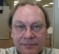## Tones in sigma delta modulator and ditheringStarted by 5 years ago12 replieslatest reply 5 years ago1216 views
Why are there tones present in 1st order sigma delta modulator and supressed in the second?
Why are there tones for DC input in sigma delta modulator?
Given Vref varying from +1 to -1, DC input = 0V, I see a peak at Fs/2 in the spectrum
How does randomly adding noise to the input signal help in overcoming tones?
[ - ]Did you look at the modulator output? 1st-order modulators produce cycles for constant-voltage input: 011011011011011011011 (for input of 2/3) or 010101010101 (for input of 1/2) or 01010110101101011010 (for input of 0.6)

In general if the input ranges from 0 to 1 and can be written as some rational number p/q then the 1st-order modulator output is periodic with period q, and therefore you'll get tones. The only way to avoid tones is for the input to be not close to rational numbers p/q with small q. (which doesn't really happen)

Adding dithering noise spreads the spectrum; you still have more or less the same energy that was present in the 1st-order modulator tones, but it is spread over a wider frequency range. Second-order and higher modulators do this automatically.

sample Python code to see this:

def dsmod(rho, n):
acc = 0
for _ in xrange(n):
acc += rho
out = 1 if acc >= 1 else 0
acc -= out
yield out
>>> list(dsmod(0.5,20))
[0, 1, 0, 1, 0, 1, 0, 1, 0, 1, 0, 1, 0, 1, 0, 1, 0, 1, 0, 1]
>>> list(dsmod(0.6,20))
[0, 1, 0, 1, 0, 1, 1, 0, 1, 0, 1, 1, 0, 1, 0, 1, 1, 0, 1, 0]
[ - ]Adding noise to any digital modulation process with large harmonic spurs on its output is effective at spreading the spurs' energy to meet FCC testing spurious output requirements. We did that at work for a VOR signal generator and it was quite effective. (One might then argue that the FCC testing requirements should rely more on averaging and not peaks, but it is what it is.)

[ - ]"(One might then argue that the FCC testing requirements should rely more on averaging and not peaks, but it is what it is.)"

One might argue in reply that in a portion of the spectrum that's assigned to traditional narrowband services, one honkin' big tone sticking up in the middle of things may not affect most users, but there'll certainly be one user who's severely affected.

I worked at a place where we had one customer who was seeing interference at one frequency from one of our microprocessor clocks.  They were big enough that we built a board just for them that had the frequency pushed over to the next-lower standard crystal frequency, with special software that loaded different values in all the relevant counters to make it work.

[ - ]You can buy spread-spectrum clocks for microprocessors and other uses just for that reason (see: http://www.ti.com/clock-and-timing/generators/spre...)

[ - ]Sir,

Thank you so much for the reply. But the doubt I have is very basic. When I have Vref ranging from -1 to 1 and input is DC 0 V, the output is 0101010101. This is passed through a Kaiser window and absolute value of FFT is taken. Here I see a spike at Fs/2. I don't understand this. When the input is 0.5V, I see 4 spikes and so on. Could you please explain this?

Attaching my code

clc;
clear all;
close all;
a=5;
Fs = 4096;
dt = 1/Fs;
t = 0:dt:1-dt;
%x = 1.5*(sin(2*pi*50.5*t))+.08*randn(size(t));

x = 0*(1+ 0*t)
l = length(x);
u(1) = 0.1
figure;
subplot(211);
plot(x,'r');
for i = 1:l
if(u(i)> 0)
y(i) = 2;
else
y(i) = -2;
end
e(i) = x(i) - y(i);
u(i+1)= u(i) + e(i);
end
subplot(212);
stairs(y,'b')
x2 = y.*kaiser(length(y),13)'
figure
plot(x2)
X1 = db(abs(fft(x)));
Y1 = db(abs(fft(x2)));
X2 = fft(x2)
%figure
%plot(X1, 'r')
figure
plot(Y1, 'b')

[ - ]You don't understand that if the time-domain output is 01010101 then you get a spike at Fs/2? Then I can't help you.
[ - ]Sir,

Basically I am new to DSP and trying to understand it through experiments. I would appreciate if you could suggest me any good material to understand this.

[ - ]To understand, forget for a moment the FFT results. Look at the signal itself and see if you understand its behavior. What is the period of the signal 0101010101010101? What about 00010001000100010001? How does this compare with the sampling time? What would you expect for frequency content?

[ - ]The period of the signal 01010101 is 2 and that of 00010001 is 4. the frequency of 010101 is twice that of 000100010001. The sampling time of 010101 is half that of 000100010001

[ - ]1.8/3 correct. (The period of the signal 01010101 is 2 samples, not just 2)  The sampling time of any discrete-time signal is just the time between individual samples, so if we're talking about the same system with different output signals then I would expect 010101 and 000100010001 and 101010010110101111101010010001 to have the same sampling time.
[ - ]You may want to start by doing some google searches of what delta sigma modulators are and how they work. A quantized signal is going to be equal to the input signal plus some error. A DS modulator acts to low pass filter the input and high pass filter the error.  However, we cannot simply filter out the error, because the output is quantized, the error has to exist.  Instead the error spectrum is shaped roughly following the shape of the high pass filter.

I’ve found lots of great information on dithering online, so don’t be shy to do some searching of your own. Basically, it’s supposed to change peaks in the error spectrum into an overall noise floor.

[ - ]